# Joins in SQL

Joins are very important in database, as all the tables are inter-related, and we need to pick records from all the related tables. Simple join between any two related or mapped table is by means of its primary – foreign keys.

For example, BOOKS and PUBLISHER tables are related by means of publisher ID in both tables. It is primary key in PUBLISHER table, whereas it is foreign key in BOOKS table. Hence these two tables are joined using publisher if and attributes from both these tables can be retrieved now to show the result. This kind of joins also makes sure that only related record values are pulled from each table.

```SELECT b.BOOK_NAME, b.ISBN, p.PUB_NAME
FROM BOOKS b, PUBLISHER p
WHERE b.PUBLISHER_ID = p.PUB_ID;```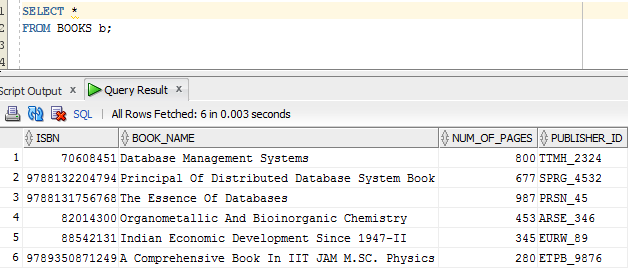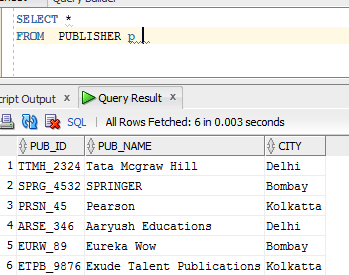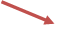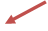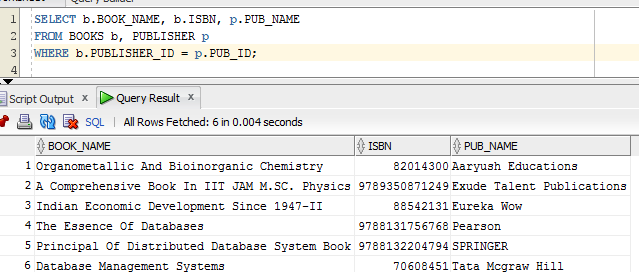## There are different types of joins available. Let us see them one by one below :

### Inner Joins

These are the normal joins between any two tables. It is also known as equijoin. That means if any two tables involved in the join has matching records, then the result is displayed. A simple example of equijoin is as discussed above. It equates the common columns to get the matching records from both the tables.

Consider another example where three tables are involved – BOOKS, BOOK_LOAN and BORROWER. These three tables are related to each other by ISBN and BRWR_ID. They can be joined to get all the required and meaningful data from the database.

```SELECT br.BRWR_NAME, b.book_name, bl.BOOK_CP_NUMBER, bl.LOAN_DATE, bl.DUE_DATE
FROM BOOKS b, BOOK_LOAN bl, BORROWER br
WHERE b.ISBN = bl.ISBN
AND bl.BRWR_ID = br.BRWR_ID;```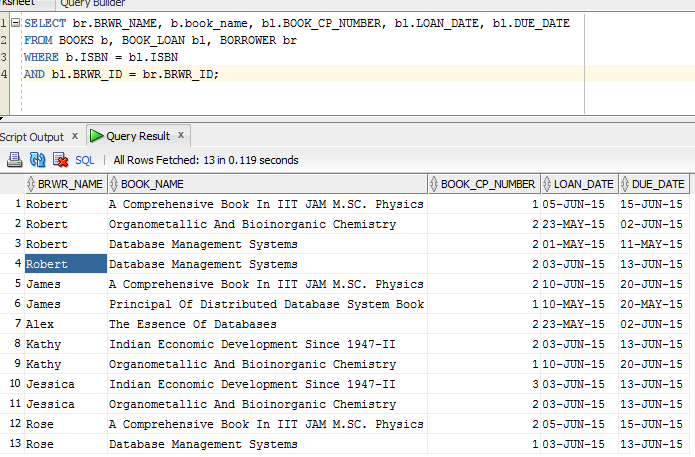### This can also be written as below : this is same as above query.

```SELECT br.BRWR_NAME,
b.book_name,
bl.BOOK_CP_NUMBER,
bl.LOAN_DATE,
bl.DUE_DATE
FROM BOOK_LOAN bl
INNER JOIN BOOKS b ON bl.ISBN = b.ISBN
INNER JOIN BORROWER br ON bl.BRWR_ID = br.BRWR_ID;```

It checks for the matching ISBN in both BOOKS and BOOK_LOAN table and retrieves those records. Similarly, for the same ISBN, it checks for the matching records in both BOOK_LOAN and BORROWER based on BRWR_ID. When matching records in all the tables are found, it displays the result.

### SELF Joins

This is same as inner join, but here instead of two different tables, same table is used join with it.
Consider a query to find the borrowers who has loaned the book that Robert has borrowed. These details are obtained from BOOK_LOAN table alone – books that Robert has borrowed are available in BOOK_LOAN table and details about other borrower are also available in BOOK_LOAN table. Hence the query to get this detail is:

```SELECT bl.ISBN AS ROBERT_BOOKS, bl_others.BRWR_ID
FROM BOOK_LOAN bl, BOOK_LOAN bl_others
WHERE bl.ISBN = bl_others.ISBN
AND bl.BRWR_ID= 'RBT_1000'
AND bl.BRWR_ID! =bl_others.BRWR_ID;```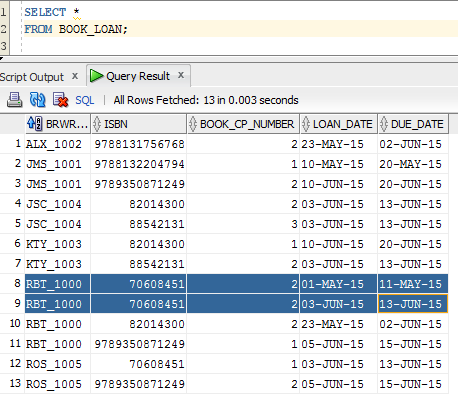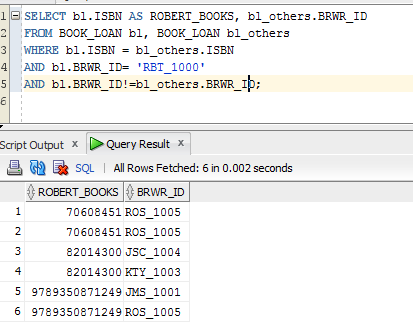Here result shows ISBN = 70608451 two times under Rose. This is because, Robert has borrowed ISBN = 70608451, two times. Hence above query executes the check two times and shows two times the result. But this is not correct as Rose has not borrowed two times. Hence we can use DISTINCT in above query to show the correct result.

```SELECT DISTINCT bl.ISBN AS ROBERT_BOOKS, bl_others.BRWR_ID
FROM BOOK_LOAN bl, BOOK_LOAN bl_others
WHERE bl.ISBN = bl_others.ISBN
AND bl.BRWR_ID= 'RBT_1000'
AND bl.BRWR_ID! =bl_others.BRWR_ID;```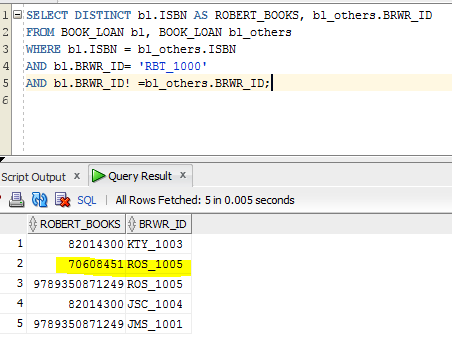### Outer Joins

These types of joins are used to show the results even if there are no matching records in other table. That means, when we perform normal joins like above, records are listed in the result, only if there is matching records exists for the columns that we join. But when outer joins are used, it checks for the matching columns in both the tables, and displays all the matching records from both the tables. In addition, it displays all the non-matching records of the table by displaying NULLs to the columns for which match is not found.

Consider below two tables AUTHOR and BOOK_AUTHOR. The table AUTHOR has all Authors’ details and BOOK_AUTHOR has all the list of books written by the Author.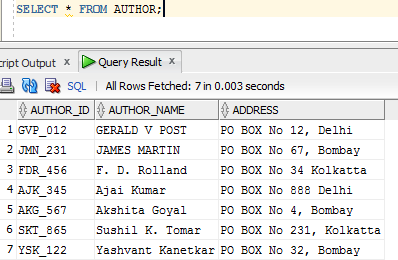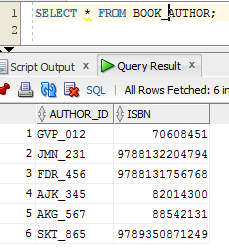Now the outer join between these two tables will find the match between both the tables and display the result. Then it will list all the records for which match is not found in either of the table and it will display NULL columns for them like below. For the author Yashvant Kanetkar, book details are not yet entered. Hence we will see NULL values for BOOK_AUTHOR details for this author.

That means, by using outer join, we can able to see all the records of the tables participating in the join irrespective of matching records. If matching records are not found in any of the table, then its columns are marked as NULL.

There are three types of outer join – left, right and full outer joins.

### LEFT Outer Joins

In this method when two tables are joined, all the records from left table are displayed. Matching records from the right table is displayed if exists, else NULLs are displayed. That means, it is a method of join where records from both the tables are not considered. When two tables joined using left outer join, only all the records from the left table are shown. It is represented by ‘LEFT OUTER JOIN’ or ‘(+)’ on the right side column in the join while using ‘=’.

```SELECT * FROM AUTHOR a
LEFT OUTER JOIN BOOK_AUTHOR ba
ON a.AUTHOR_ID = ba.AUTHOR_ID;```

OR
```SELECT *
FROM AUTHOR a, BOOK_AUTHOR ba
WHERE a.AUTHOR_ID = ba.AUTHOR_ID (+);```

Here AUTHOR is the left table and BOOK_AUTHOR is the right table. When joined using ‘LEFT OUTER JOIN’ clause, we use ‘ON’ to have the joining condition. Here both the tables are joined using AUTHOR_ID. We can have other conditions / filters on the tables, which can be listed under ‘WHERE’ clause. Only the joining conditions for the tables participating in left outer join are listed in ‘ON’ clause.

When ‘(+)’ notation is used, we have to use it on the right hand side column of the ‘=’ sign to indicate that all the records from the left side table has to be shown.

This query will search all the matching records from both the tables based on a.AUTHOR_ID = ba.AUTHOR_ID.  Then it will list all the unmatched records from left table – AUTHOR and make the columns of right table as NULL. We can see here that Yashvant does not have any books in BOOK_AUTHOR table and hence those details are marked as NULL when joined. If it was a normal join, then we wouldn’t have had this record at all.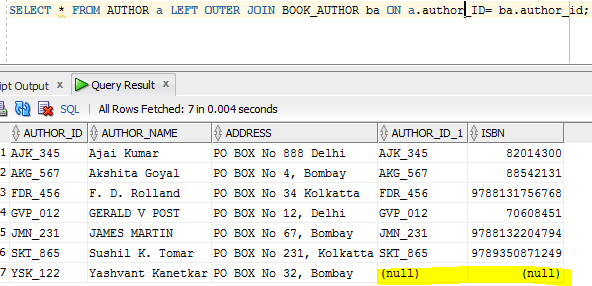We can have any other conditions / filters to the query in the WHERE clause.

```SELECT * FROM AUTHOR a
LEFT OUTER JOIN BOOK_AUTHOR ba
ON a.AUTHOR_ID = ba.AUTHOR_ID
WHERE a.AUTHOR_NAME LIKE ‘A%’;```

OR
```SELECT *
FROM AUTHOR a, BOOK_AUTHOR ba
WHERE a.AUTHOR_ID = ba.AUTHOR_ID (+)
AND a.AUTHOR_NAME LIKE ‘A%’;```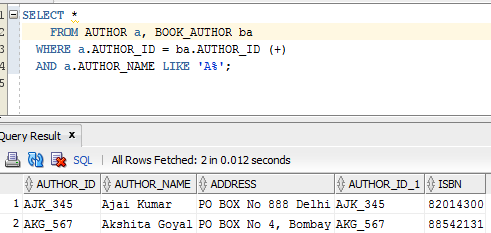Let us consider another example with BOOK_COPY and BOOK_LOAN. Here it has listed all the records from left table – BOOK_COPY is listed first. Non matching record has NULLs on the right hand columns.

```SELECT * FROM BOOK_COPY bc
LEFT OUTER JOIN BOOK_LOAN bl
ON bc.ISBN = bl.ISBN AND bc.COPY_NUM = bl.BOOK_CP_NUMBER;```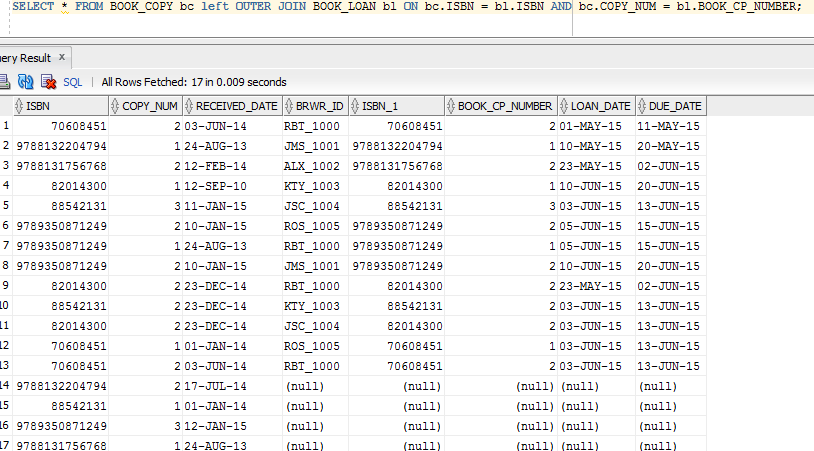### RIGHT Outer Joins

This is opposite of Left Outer Join.  Here all records from right table is displayed while NULLs are displayed for the records that are not found in the left table. It is represented by either ‘RIGHT OUTER JOIN’ clause or ‘(+)’ on the left side column while ‘=’ symbol is used.

```SELECT * FROM BOOK_AUTHOR ba
RIGHT OUTER JOIN AUTHOR a – here order of tables important
ON ba.AUTHOR_ID = a.AUTHOR_ID;```

OR
```SELECT *
FROM BOOK_AUTHOR ba, AUTHOR a
WHERE ba.AUTHOR_ID (+) = a.AUTHOR_ID; -- here position of (+) is important```

Here BOOK_AUTHOR is the left table and AUTHOR is the right table. When right outer join is used, all the records from the AUTHOR are listed along with matching records from BOOK_AUTHOR. For the records for which matching records are not found in BOOK_AUTHOR, NULLs are displayed in the result set.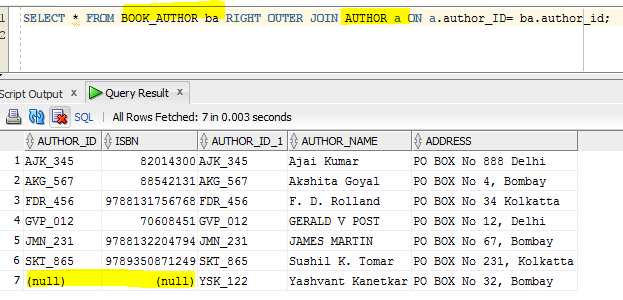Like left outer join, we can have any other filters on the query using WHERE clause.

```SELECT * FROM BOOK_AUTHOR ba
RIGHT OUTER JOIN AUTHOR a
ON ba.AUTHOR_ID = a.AUTHOR_ID

OR
```SELECT *
FROM BOOK_AUTHOR ba, AUTHOR a
WHERE ba.AUTHOR_ID (+) = a.AUTHOR_ID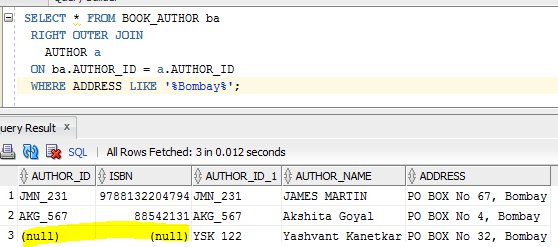Though we said position is important above, consider the below query on BOOK_COPY and BOOK_LOAN to see how it makes the difference. In the above query, we had parent – child mapping and hence we did not have any non matching columns in the BOOK_AUTHOR. Hence to show right outer join on the same tables that are participated in the left outer join, position of the table had taken importance. But in below case even though we have mappings, we did not create any constraint on copy number. Hence we have non matching records in both the tables. Thus below example shows how left and right outer join is different without changing the positions of the table. If we change left to right in the example of left outer join above, we get different results here. It has listed all the records from right table – BOOK_LOAN and NULLs to the non matching records in left table – BOOK_COPY.

```SELECT * FROM BOOK_COPY bc
RIGHT OUTER JOIN BOOK_LOAN bl
ON bc.ISBN = bl.ISBN AND bc.COPY_NUM = bl.BOOK_CP_NUMBER;```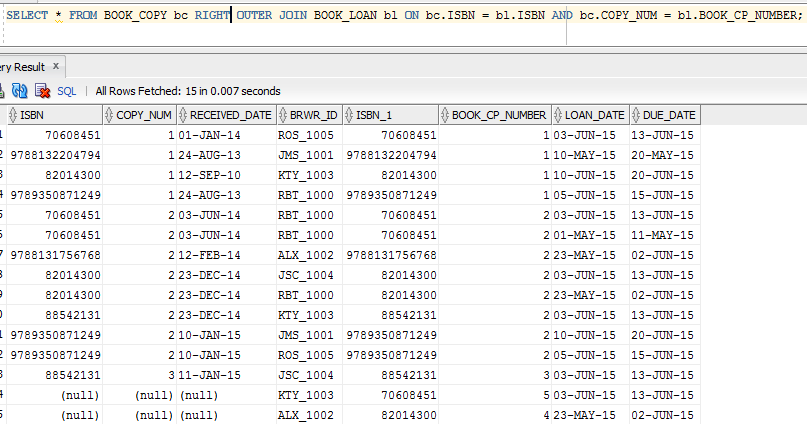### FULL Outer Joins

This is combination of both left and right outer join. Here records from both the tables are listed. If matching records are found, then those records are displayed. If no matching records are found then they are displayed as NULL. It is denoted by ‘FULL OUTER JOIN’.

Here BOOK_COPY is the left table and BOOK_LOAN is the right table. When full outer join is used, it will first list all the records from left table- BOOK_COPY. Then it will take ALL the records from right table BOOK_LOAN, and check if any matching record exists in it for the left table records. If exists, it will display that record against left table record, else put NULLs for those left table record and continue with rest of the record. If it does not find records in left table, then it will enter the right table records with NULL records on the left side columns.

In the below result, we can see that it has listed all the records from BOOK_COPY and BOOK_LOAN first. Then it has checked for the matching records in both the tables and mapped with each other to display them. When matching records are not found in any of the table, then it has marked it as NULL irrespective of which side. i.e.; it has result of both left and right outer joins!

```SELECT * FROM BOOK_COPY bc
FULL OUTER JOIN BOOK_LOAN bl
ON bc.ISBN = bl.ISBN AND bc.COPY_NUM = bl.BOOK_CP_NUMBER;```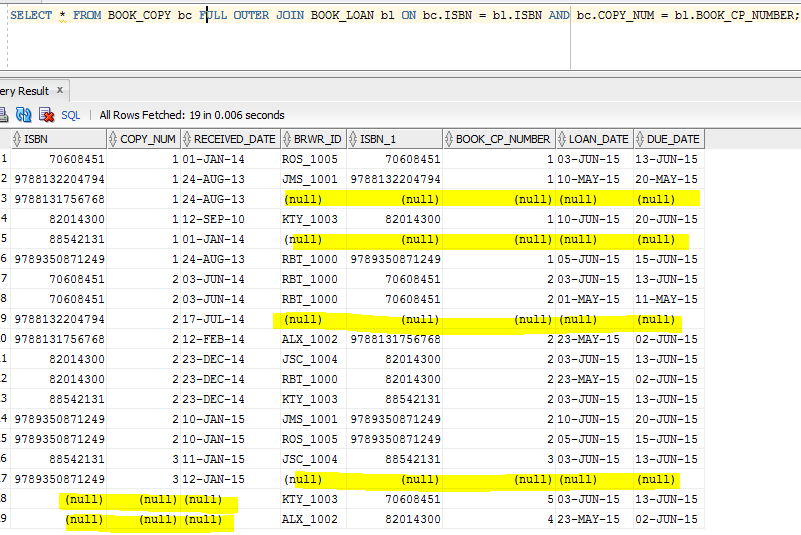We can have more than one table participating in the outer joins like below. But we cannot cross join the tables- that means one table should always remain as left or right table and it can have any number of right or left tables. In such case we can have left, right or full outer join.

```SELECT * FROM BOOK_AUTHOR ba
FULL OUTER JOIN BOOKS b ON ba.ISBN = b.ISBN
FULL OUTER JOIN AUTHOR a ON ba.author_id = a.author_id;```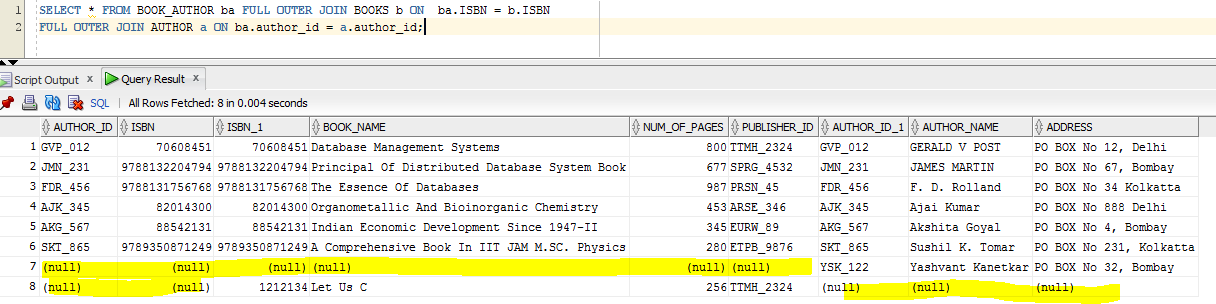### Cartesian Joins

This is the join which combines both the table record, irrespective of any keys or join conditions. Here each record of one table is mapped with entire records in the other table. Consider the below query on BOOKS and AUTHOR. We have not specified any joining condition. Hence it maps all the books in BOOKS table to the authors in the BOOK_AUTHOR table. We can see below that for the same ISBN from BOOKS table we have different ISBNs mapped. This indicates that records are cross joined with each other. For such joins, we need not have any mappings or parent-child relationship.

`SELECT * FROM BOOKS b, BOOK_AUTHOR ba;`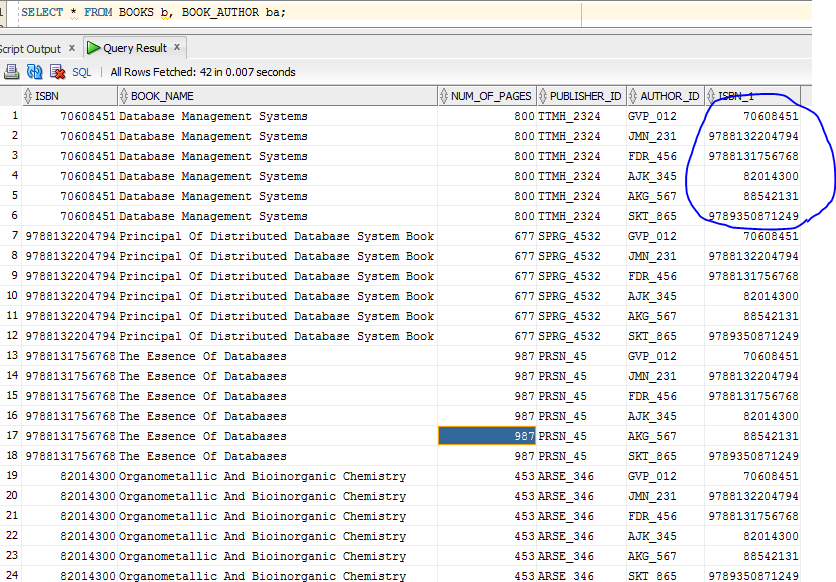Translate »